# HashMap详解

352 篇文章 0 订阅

### 什么是HashMap?

HashMap是基于哈希表的Map接口的非同步实现。此实现提供所有可选的映射操作，并允许使用null值和null键。此类不保证映射的顺序，特别是它不保证该顺序恒久不变。

HashMap的数据结构 在Java编程语言中，最基本的结构就是两种，一个是数组，另外一个是模拟指针（引用），所有的数据结构都可以用这两个基本结构来构造的，HashMap也不例外。HashMap实际上是一个“链表散列”的数据结构，即数组和链表的结合体。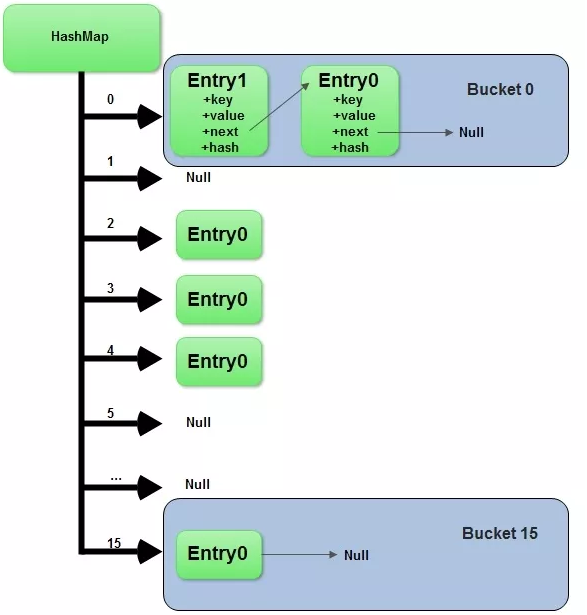1. public class HashMap<K,V> extends AbstractMap<K,V>

2.    implements Map<K,V>, Cloneable, Serializable {

3.

4.    // 默认容量，默认为16，必须是2的幂

5.    static final int DEFAULT_INITIAL_CAPACITY = 1 << 4;

6.

7.    // 最大容量，值是2^30

8.    static final int MAXIMUM_CAPACITY = 1 << 30

9.

10.    // 装载因子，默认的装载因子是0.75

11.    static final float DEFAULT_LOAD_FACTOR = 0.75f;

12.

13.    // 解决冲突的数据结构由链表转换成树的阈值，默认为8

14.    static final int TREEIFY_THRESHOLD = 8;

15.

16.    // 解决冲突的数据结构由树转换成链表的阈值，默认为6

17.    static final int UNTREEIFY_THRESHOLD = 6;

18.

19.    /* 当桶中的bin被树化时最小的hash表容量。

20.     *  如果没有达到这个阈值，即hash表容量小于MIN_TREEIFY_CAPACITY，当桶中bin的数量太多时会执行resize扩容操作。

21.     *  这个MIN_TREEIFY_CAPACITY的值至少是TREEIFY_THRESHOLD的4倍。

22.     */

23.    static final int MIN_TREEIFY_CAPACITY = 64;

24.

25.    static class Node<K,V> implements Map.Entry<K,V> {

26.        //...

27.    }

28.    // 存储数据的数组

29.    transient Node<K,V>[] table;

30.

31.    // 遍历的容器

32.    transient Set<Map.Entry<K,V>> entrySet;

33.

34.    // Map中KEY-VALUE的数量

35.    transient int size;

36.

37.    /**

38.     * 结构性变更的次数。

39.     * 结构性变更是指map的元素数量的变化，比如rehash操作。

40.     * 用于HashMap快速失败操作，比如在遍历时发生了结构性变更，就会抛出ConcurrentModificationException。

41.     */

42.    transient int modCount;

43.

44.    // 下次resize的操作的size值。

45.    int threshold;

46.

47.    // 负载因子，resize后容量的大小会增加现有size * loadFactor

48.    final float loadFactor;

49. }

### HashMap的初始化

 
1. public HashMap() {

2.        this.loadFactor = DEFAULT_LOAD_FACTOR; // 其他值都是默认值

3.    }

### HashMap的存储操作

 
1. public V put(K key, V value) {

2.        return putVal(hash(key), key, value, false, true);

3.    }

### hash计算，确定数组索引位置

 
1. static final int hash(Object key) {   //jdk1.8

2.     int h;

3.     // h = key.hashCode() 为第一步 取hashCode值

4.     // h ^ (h >>> 16)  为第二步 高位参与运算

5.     return (key == null) ? 0 : (h = key.hashCode()) ^ (h >>> 16);

6. }

 
1. bucketIndex = indexFor(hash, table.length);

2. //indexFor的代码也很简单，就是把散列值和数组长度做一个"与"操作，

3. static int indexFor(int h, int length) {

4.   return h & (length-1);

5. }

 
1. 10100101 11000100 00100101

2. & 00000000 00000000 00001111

3. ----------------------------------

4.  00000000 00000000 00000101 //高位全部归零，只保留末四位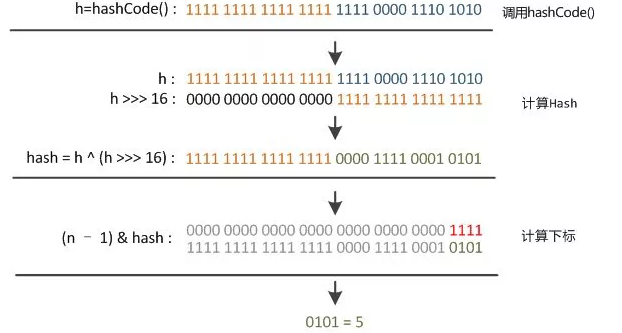### putVal方法

HashMap的put方法执行过程可以通过下图来理解，自己有兴趣可以去对比源码更清楚地研究学习。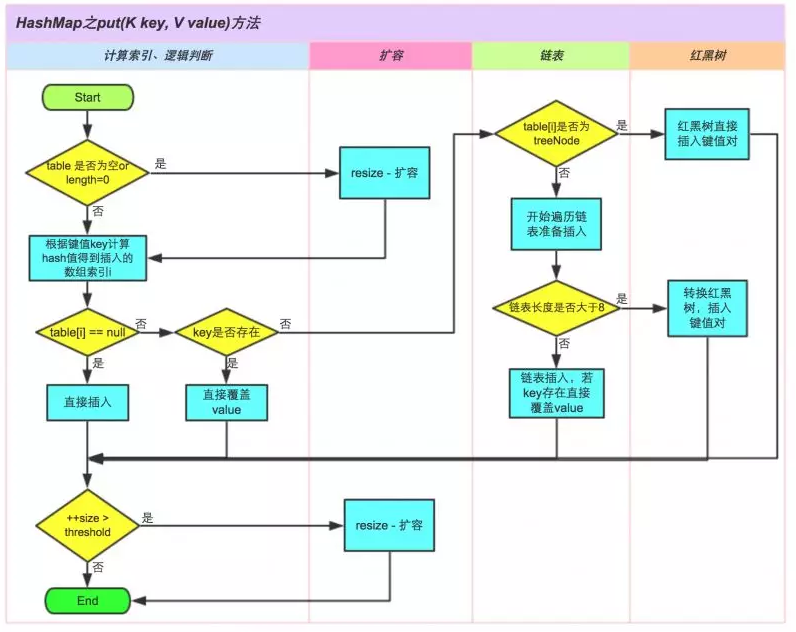1. // 真正的put操作

2.    final V putVal(int hash, K key, V value, boolean onlyIfAbsent,

3.                   boolean evict) {

4.        Node<K,V>[] tab; Node<K,V> p; int n, i;

5.        // 如果table没有初始化，或者初始化的大小为0，进行resize操作

6.        if ((tab = table) == null || (n = tab.length) == 0)

7.            n = (tab = resize()).length;

8.        // 如果hash值对应的桶内没有数据，直接生成结点并且把结点放入桶中

9.        if ((p = tab[i = (n - 1) & hash]) == null)

10.            tab[i] = newNode(hash, key, value, null);

11.        // 如果hash值对应的桶内有数据解决冲突，再放入桶中

12.        else {

13.            Node<K,V> e; K k;

14.            //判断put的元素和已经存在的元素是相同(hash一致，并且equals返回true)

15.            if (p.hash == hash &&

16.                ((k = p.key) == key || (key != null && key.equals(k))))

17.                e = p;

18.            // put的元素和已经存在的元素是不相同(hash一致，并且equals返回true)

19.            // 如果桶内元素的类型是TreeNode，也就是解决hash解决冲突用的树型结构，把元素放入树种

20.            else if (p instanceof TreeNode)

21.                e = ((TreeNode<K,V>)p).putTreeVal(this, tab, hash, key, value);

22.            else {

23.                // 桶内元素的类型不是TreeNode，而是链表时，把数据放入链表的最后一个元素上

24.                for (int binCount = 0; ; ++binCount) {

25.                    if ((e = p.next) == null) {

26.                        p.next = newNode(hash, key, value, null);

27.                        // 如果链表的长度大于转换为树的阈值(TREEIFY_THRESHOLD)，将存储元素的数据结构变更为树

28.                        if (binCount >= TREEIFY_THRESHOLD - 1) // -1 for 1st

29.                            treeifyBin(tab, hash);

30.                        break;

31.                    }

32.                    // 如果查已经存在key，停止遍历

33.                    if (e.hash == hash &&

34.                        ((k = e.key) == key || (key != null && key.equals(k))))

35.                        break;

36.                    p = e;

37.                }

38.            }

39.            // 已经存在元素时

40.            if (e != null) { // existing mapping for key

41.                V oldValue = e.value;

42.                if (!onlyIfAbsent || oldValue == null)

43.                    e.value = value;

44.                afterNodeAccess(e);

45.                return oldValue;

46.            }

47.        }

48.        ++modCount;

49.        // 如果K-V数量大于阈值，进行resize操作

50.        if (++size > threshold)

51.            resize();

52.        afterNodeInsertion(evict);

53.        return null;

54.    }

### 扩容机制

HashMap的扩容机制用的很巧妙，以最小的性能来完成扩容。 扩容后的容量就变成了变成了之前容量的2倍，初始容量为16，所以经过rehash之后，元素的位置要么是在原位置，要么是在原位置再向高下标移动上次容量次数的位置，也就是说如果上次容量是16，下次扩容后容量变成了16+16，如果一个元素在下标为7的位置，下次扩容时，要不还在7的位置，要不在7+16的位置。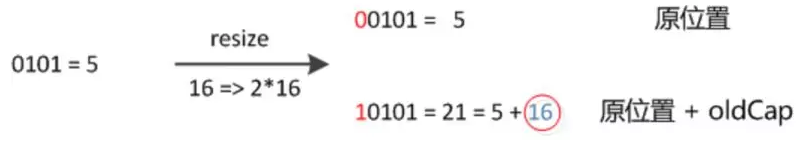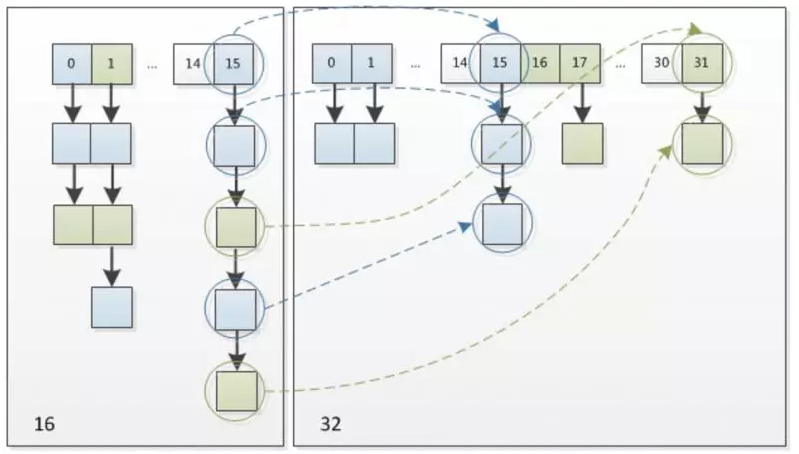1. final Node<K,V>[] resize() {

2.        Node<K,V>[] oldTab = table;

3.        int oldCap = (oldTab == null) ? 0 : oldTab.length;

4.        int oldThr = threshold;

5.        int newCap, newThr = 0;

6.        // 计算新的容量值和下一次要扩展的容量

7.        if (oldCap > 0) {

8.        // 超过最大值就不再扩充了，就只好随你碰撞去吧

9.            if (oldCap >= MAXIMUM_CAPACITY) {

10.                threshold = Integer.MAX_VALUE;

11.                return oldTab;

12.            }

13.            // 没超过最大值，就扩充为原来的2倍

14.            else if ((newCap = oldCap << 1) < MAXIMUM_CAPACITY &&

15.                     oldCap >= DEFAULT_INITIAL_CAPACITY)

16.                newThr = oldThr << 1; // double threshold

17.        }

18.        else if (oldThr > 0) // initial capacity was placed in threshold

19.            newCap = oldThr;

20.        else {               // zero initial threshold signifies using defaults

21.            newCap = DEFAULT_INITIAL_CAPACITY;

22.            newThr = (int)(DEFAULT_LOAD_FACTOR * DEFAULT_INITIAL_CAPACITY);

23.        }

24.        // 计算新的resize上限

25.        if (newThr == 0) {

26.            float ft = (float)newCap * loadFactor;

27.            newThr = (newCap < MAXIMUM_CAPACITY && ft < (float)MAXIMUM_CAPACITY ?

28.                      (int)ft : Integer.MAX_VALUE);

29.        }

30.        threshold = newThr;

31.        @SuppressWarnings({"rawtypes","unchecked"})

32.            Node<K,V>[] newTab = (Node<K,V>[])new Node[newCap];

33.        table = newTab;

34.        if (oldTab != null) {

35.            // 把每个bucket都移动到新的buckets中

36.            for (int j = 0; j < oldCap; ++j) {

37.                Node<K,V> e;

38.                //如果位置上没有元素，直接为null

39.                if ((e = oldTab[j]) != null) {

40.                    oldTab[j] = null;

41.                    //如果只有一个元素，新的hash计算后放入新的数组中

42.                    if (e.next == null)

43.                        newTab[e.hash & (newCap - 1)] = e;

44.                    //如果是树状结构，使用红黑树保存

45.                    else if (e instanceof TreeNode)

46.                        ((TreeNode<K,V>)e).split(this, newTab, j, oldCap);

47.                    //如果是链表形式

48.                    else { // preserve order

49.                        Node<K,V> loHead = null, loTail = null;

50.                        Node<K,V> hiHead = null, hiTail = null;

51.                        Node<K,V> next;

52.                        do {

53.                            next = e.next;

54.                            //hash碰撞后高位为0，放入低Hash值的链表中

55.                            if ((e.hash & oldCap) == 0) {

56.                                if (loTail == null)

57.                                    loHead = e;

58.                                else

59.                                    loTail.next = e;

60.                                loTail = e;

61.                            }

62.                            //hash碰撞后高位为1，放入高Hash值的链表中

63.                            else {

64.                                if (hiTail == null)

65.                                    hiHead = e;

66.                                else

67.                                    hiTail.next = e;

68.                                hiTail = e;

69.                            }

70.                        } while ((e = next) != null);

71.                        // 低hash值的链表放入数组的原始位置

72.                        if (loTail != null) {

73.                            loTail.next = null;

74.                            newTab[j] = loHead;

75.                        }

76.                        // 高hash值的链表放入数组的原始位置 + 原始容量

77.                        if (hiTail != null) {

78.                            hiTail.next = null;

79.                            newTab[j + oldCap] = hiHead;

80.                        }

81.                    }

82.                }

83.            }

84.        }

85.        return newTab;

86.    }

12-301913
12-0912112-042420
06-08388
04-181557
10-2467
08-06916
12-0779
11-221万+
01-031093
08-02160
03-191902
03-15269
06-0142
11-27136
11-0450
01-1697
09-01283
06-2519点击重新获取扫码支付余额充值